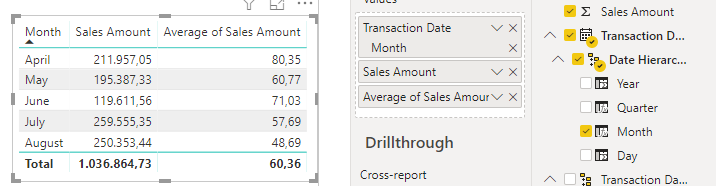cancel
Showing results for
Did you mean:Anonymous
Not applicable

## Average by month

Hi, when calculating the monthly sales average, shouldn't it be calculated as total sales amount / number of days? why is powerBI calculating this way?2 ACCEPTED SOLUTIONSResponsive Resident

Hello,

By default, it will put the average sales amount of that month, if you want to use the diary monthly average, you will have to create a measure to sum the monthly sales, and then, divide it by the number of days.Community Support

Hi @Anonymous ,

In addition, if you need to calculate the average by day, you can create measure using codes below .

`Average by day= DIVIDE(SUM([Sale Amount]),CALCULATE(COUNT(Table1[Date]), FILTER(ALLSELECTED(Table1), YEAR(Table1[Date]) =YEAR(MAX(Table1[Date]))&&MONTH(Table1[Date]) =MONTH(MAX(Table1[Date])))))`

Best Regards,

Amy

If this post helps, then please consider Accept it as the solution to help the other members find it more quickly.

3 REPLIES 3Community Support

Hi  ,

Does that make sense? If so, kindly mark the proper reply as a solution to help others having the similar issue and close the case. If not, let me know and I'll try to help you further.

Best regards

AmyCommunity Support

Hi @Anonymous ,

In addition, if you need to calculate the average by day, you can create measure using codes below .

`Average by day= DIVIDE(SUM([Sale Amount]),CALCULATE(COUNT(Table1[Date]), FILTER(ALLSELECTED(Table1), YEAR(Table1[Date]) =YEAR(MAX(Table1[Date]))&&MONTH(Table1[Date]) =MONTH(MAX(Table1[Date])))))`

Best Regards,

Amy

If this post helps, then please consider Accept it as the solution to help the other members find it more quickly.Responsive Resident

Hello,

By default, it will put the average sales amount of that month, if you want to use the diary monthly average, you will have to create a measure to sum the monthly sales, and then, divide it by the number of days.